# Physics - Electromagnetism - Lenz's law and Induced Electric fields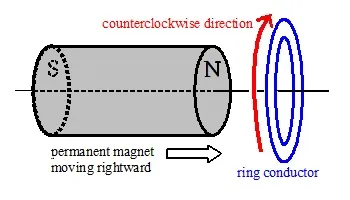Image source:## Introduction

Hello it's a me again Drifter Programming! Today we continue with Electromagnetism to get into Lenz's law and also Induced Electric Fields. So, without further do, let's get straight into it!

## Lenz's law

In the previous post we got into how we find the direction of the induced electric current using an "physical" explanation based on the magnetic and electric forces that act on charges and current. Another much simpler way of finding the direction of the induced current is using Lenz's law. Lenz's law states that "The induced current flows in such a direction so that it opposes the changes that induced it".

For example when moving a permanent bar magnet through a coil of wire an electric current is induced in the coil. As we know from electric field sources, this current sets up a magnetic field around the coil, making it a magnet. Using Lenz's law we can indicate the direction of the induced current. Like poles repel each other and so when the north pole of the bar magnet is approaching the coil, the induced current flows in such a way as to make the side of the coil that is being approached a north pole too. When withdrawing the magnet again the near side of the coil becomes a south pole so that it produces an attractive force (opposite poles), which opposes the change again.

From Lenz's law, which is a law based on Faraday's law, we can now understand the negative sign (-) used in Faraday's law much much better. The change in magnetic flux (dΦ) and induced emf (ε) have opposite signs, cause the induced emf wants to oppose the effect of the magnetic flux change. That's why Faraday's law is written as: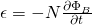where:

• ε is the induced emf
• Φ the magnetic flux (partial derivative by time)
• N the number of turns in the coil

Lenz's law is also obeying the conservation of energy! The direction of the magnetic field that creates the induced current and the magnetic field of the current in a conductor are stated to not be in the same direction. If they where in the same direction then these two magnetic fields would add up and produce current of twice the magnitude and so would create a stronger magnetic fieled, which would cause more current and so on. We can clearly see that this last thing would lead into the violation of the law of conservaton of energy, cause voltage/current cannot be created or destroyed!

Also note that the induced current creates a magnetic field which is equal and opposite to the direction of the magnetic field that creates it. This obeys Newton's third law of motion (action-reaction), cause the induced current resists the change in the magnetic field in the area. To find the direction of the magnetic field or current we still use the same right hand thumb rule as before: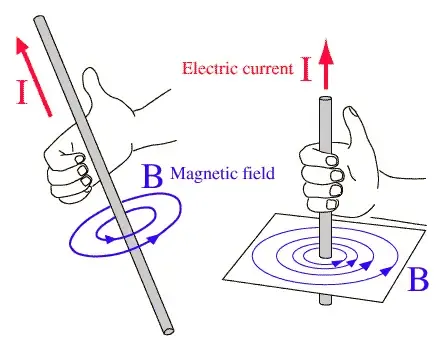So, to summarize really quick:

• The direction of the induced current in the coil will be such that is opposes the change (increase or decrease) of flux caused by a magnet in the area of the conductor.
• The direction can be found by using the right hand thumb rule.

## Induced Electric Fields

The emf's that are induced in the circuit imply that some work is being done. The work done by the electric field E for each unit of charge completely around a circuit gives us the induced emf ε as: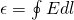Using this intergral Faraday's law can be written in terms of the induced electric field as: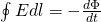Wait....isn't the left part always zero? Well, for Electrostatic Electric fields which are caused by fixed charge distributions we of course know that there is no net work over a closed path which means that the integral gives us zero. But something that is important to distinguish is that Induced Electric Fields don't obey this, which means that the result of the integral is non-zero and so electric potential can only be associated with electrostatic fields, but not with induced fields. Remember that induced electric fields and electrostatic fields are different from each other. An electrostatic field is produced by a static distribution of charge and determined via Coulomb's law (and Law's that are based on it). An induced electric field is a nonconservative electric field and produced by a changing magnetic field (magnetic flux).

Note that the integral form of Fraday's law is valid even if no conductor is present in the region.

### Small example

Let's get into a small example. Consider a uniform magnetic field confined in a circular region with radius R, on top of a page. Suppose that the magnitude of B increases with time (dB/dT >0). Can we find the induced electric field everywhere?

The magnetic field is confined to a circular region and so for symmetry we choose the integration path to be a circle of radius r. The magnitude of the induced electric field at all points on a circle is the same and so according to Lenz's law the direction of E must be such that it would drive the induced current to produce a magnetic field that opposes the change in magnetic flux. The area vector is pointing out of the page and the magnetic flux is negative or inward. The inward magnetic flux is increasing and therefore the induced current flows in a counterclockwise motion to produce more outward flux.

For r < R we get that: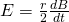which can be found easily, cause the area of the region is πr^2

For r > R we get: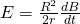cause the calculation process now contains both r and R and so R doesn't get cutoff like before...

### REFERENCES:

Mathematical equations that I had in this post where drawn using quicklatex

### Electric fields:

Getting into Electromagnetism -> electromagnetim, electric charge, conductors, insulators, quantization

Coulomb's law with examples -> Coulomb's law, superposition principle, Coulomb constant, how to solve problems, examples

Electric fields and field lines -> Electric fields, Solving problems around Electric fields and field lines

Electric dipoles -> Electric dipole, torque, potential and field

Electric charge and field Exercises -> examples in electric charges and fields

### Electric flux:

Electric flux and Gauss's law -> Electric flux, Gauss's law

Applications of Gauss's law (part 1) -> applying Gauss's law, Gauss applications

Applications of Gauss's law (part 2) -> more Gauss applications

Electric flux exercises -> examples in electric flux and Gauss's law

### Electric potential:

Electric potential energy -> explanation of work-energy, electric potential energy

Calculating electric potentials -> more stuff about potential energy, potential, calculating potentials

Millikan's Oil Drop Experiment -> Millikan's experiment, electronvolt

Cathode ray tubes explained using electric potential -> cathode ray tube explanation

Electric potential exercises (part 1) -> applications of potential

Electric potential exercises (part 2) -> applications of potential gradient, advanced examples

### Capacitance:

Capacitors (Condensers) and Capacitance -> Capacitors, capacitance, calculating capacitance

How to solve problems around Capacitors -> combination, solving problems, simple example

Electric field energy and density -> Electric field energy, energy density

Dielectric materials -> Dielectrics, dielectric constant, permittivity and strength, how to solve problems

Electric capacitance exercises -> examples in capacitance, energy density and dielectrics

### Current, resistance and EMF:

Electric current -> Electric current, current density

Electrical resistivity and conductivity -> Electrical resistivity, conductivity, thermal coefficient of resistivity, hyperconductivity

Electric resistance -> Resistance, temperature, resistors

Electromotive Force (EMF) and Internal resistance -> Electromotive force, internal resistance

Power and Wattage of Electronic Circuits -> Power in general, power/wattage of electronic circuits

Electric current, resistance and emf exercises -> exampes in all those topics

### Direct current (DC) circuits:

Resistor Combinations -> Resistor combinations, how to solve problems

Kirchhoff's laws with applications -> Kirchhoff's laws, how to solve problems, applications

Electrical measuring instruments -> what are they?, types list, getting into some of them, an application

Electronic circuits with resistors and capacitors (R-C) -> R-C Circuit, charging, time constant, discharging, how to apply

RC circuit exercises -> examples in Kirchhoff, charging, discharging capacitor with/without internal resistance

### Magnetic field and forces:

Magnetic fields -> Magnetism, Magnetic field

Magnetic field lines and Gauss's law of Magnetism -> magnetic field lines, mono- and dipoles, Flux, Gauss's law of magnetism

The motion of charged particles inside of a magnetic field -> straight-line, spiral and helical particle motion

Applications of charged particle motion -> CERN, Cyclotrons, Synchrotrons, Cavity Magetron, Mass Spectrometry and Magnetic lens

Magnetic force applied on Current-Carrying Conductors -> magnetic force on current-carrying conductors/wires, proofs

Magnetic force and torque applied on current loops (circuits) -> magnetic force on current loops, magnetic moment and torque

Explaining the Physics behind Electromotors -> tesla, history and explaining the physics behind them

Magnetic field exercises -> examples in magnetic force, magnetic flux, particle motion and forces/torque on current-carrying conductors

### Magnetic field sources:

Magnetic field of a moving charged particle -> moving charge, magnetic field, force between parallel charged particles

Magnetic field of current-carrying conductors -> magnetic field of current, Biot-Savart law

Force between parallel conductors and the magnetic field of a current loop-> force between parallel conductors, magnetic field of current loop

Ampere's law and Applications -> Ampere's law, applications

Magnetic materials -> Magnetic materials, classification and types, material examples

Displacement current -> Displacement current, Extension of Ampere's law

Exercises in Magnetic field sources -> examples all around magnetic field sources

### Electromagnetic Induction:

Motional Electromotive Force (Emf) -> Motional Emf, Faraday's law and motional emf, generalization

And this is actually it for today's post! Next time in Physics we will get into Εddy currents and after that I'm thinking of getting into Maxwell equations as a whole, which might be an interesting article!

C ya!

H2
H3
H4
3 columns
2 columns
1 column